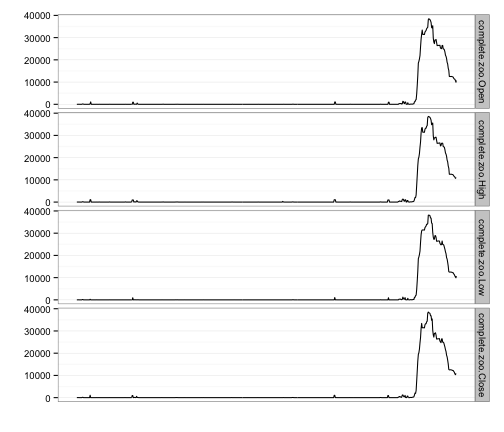# R : Irregular Time Series To Hourly Summary

This bit of R takes an irregular time series generated by attempting to scrape data every 15 minutes from Central Maine Power's web site and then converts it to a regular 15 min interval series and then aggregates it into an hourly time series for (eventual) use with this.

First, we load some necessary timey-wimey packages:

``````library(zoo)
library(chron)
library(xts)
``````

Then we read in one of the irregular time series data sets and strip off seconds and milliseconds (since they aren't useful or helpful for the rest of the operations).

``````outage.raw <- read.csv("http://rud.is/outage/data/KENNEBEC.csv", stringsAsFactors = FALSE)
outage.raw\$ts <- as.POSIXct(gsub("\\:[0-9]+\\..*\$", "", outage.raw\$ts), format = "%Y-%m-%d %H:%M")
str(outage.raw)
``````
``````## 'data.frame':    837 obs. of  2 variables:
##  \$ ts          : POSIXct, format: "2013-11-28 03:15:00" "2013-11-28 03:30:00" ...
##  \$ withoutPower: int  2 4 4 4 18 18 18 18 18 18 ...
``````

Now, we convert it to a zoo object and fill in that `zoo` object since `NAs` will cause issues

``````outage.zoo <- zoo(outage.raw\$withoutPower, outage.raw\$ts)

complete.zoo <- merge(outage.zoo, zoo(, seq(start(outage.zoo), end(outage.zoo),
by = "15 min")), all = TRUE)
complete.zoo[is.na(complete.zoo)] <- 0
str(complete.zoo)
``````
``````## 'zoo' series from 2013-11-28 03:15:00 to 2013-12-26 17:15:00
##   Data: num [1:2745] 2 4 4 4 18 18 18 18 18 18 ...
##   Index:  POSIXct[1:2745], format: "2013-11-28 03:15:00" "2013-11-28 03:30:00" ...
``````

Finally, we aggregate the data to hourly readings and trim the series down to the last 30 days:

``````hourly.zoo <- last(to.hourly(complete.zoo), "30 days")
str(hourly.zoo)
``````
``````## 'zoo' series from 2013-11-28 03:45:00 to 2013-12-26 17:15:00
##   Data: num [1:687, 1:4] 2 4 18 18 18 14 0 0 0 0 ...
##  - attr(*, "dimnames")=List of 2
##   ..\$ : NULL
##   ..\$ : chr [1:4] "complete.zoo.Open" "complete.zoo.High" "complete.zoo.Low" "complete.zoo.Close"
##   Index:  POSIXct[1:687], format: "2013-11-28 03:45:00" "2013-11-28 04:45:00" ...
``````

Here's a quick graphical overview of the final product

``````plot(hourly.zoo)
``````Or, if you prefer `ggplot`:

``````library(reshape2)
library(ggplot2)
library(scales)

df <- data.frame(hourly.zoo)
df\$ts <- as.POSIXct(rownames(df), format = "%Y-%m-%d %H:%M:%S")
df <- melt(df, c("ts"))
``````
``````gg <- ggplot(df, aes(x = ts, y = value))
gg <- gg + geom_line()
gg <- gg + scale_x_datetime(breaks = NULL)
gg <- gg + labs(x = "", y = "")
gg <- gg + facet_grid(variable ~ .)
gg <- gg + theme_bw()
gg <- gg + theme(axis.text.x = element_blank(), axis.ticks.x = element_blank())
print(gg)
``````